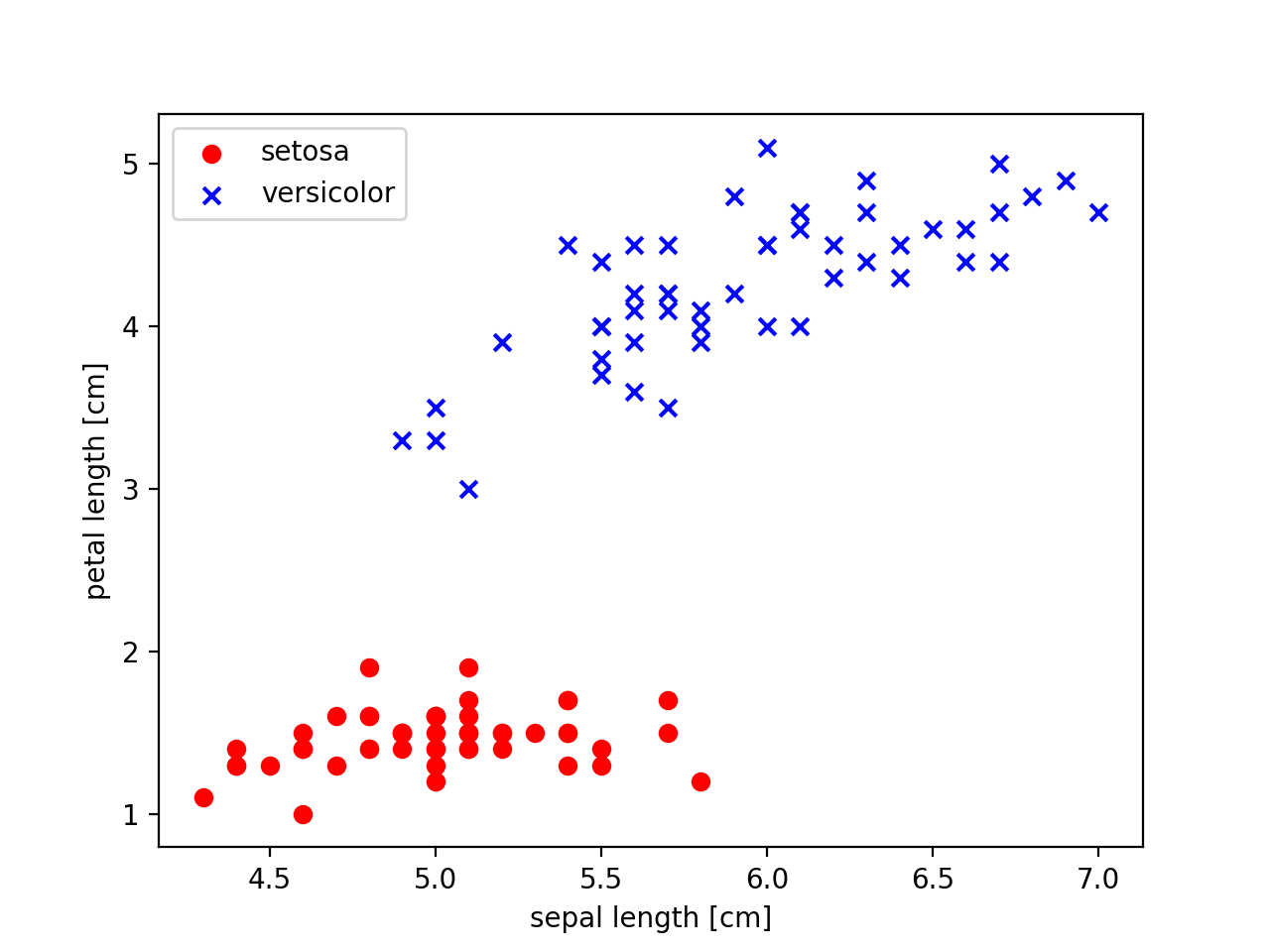[PYTHON] Data set for machine learning

What This is an article that visualizes a dataset for implementing the Perceptron model, which is a rudimentary model of machine learning.

Content

Dataset visualization with Numpy Pandas Matplot

What is the Perceptron model? Will not be mentioned here. It's a famous model, so if you look it up, you'll find a lot. This is the first model I coded since I started studying machine learning.

Is the dataset used this time an institution called ** UCI Machine Learning Repository **? Or or a kind of open source to flower dataset Iris is used as an example.

First, check the dataset. Get the dataset online and view its contents using the os module and the pandas library.

import os
import pandas as pd

s = os.path.join('https://archive.ics.uci.edu', 'ml', 'machine-learning-databases', 'iris', 'iris.data')
print(df)

The result of executing the above code is

0    1    2    3               4
0    5.1  3.5  1.4  0.2     Iris-setosa
1    4.9  3.0  1.4  0.2     Iris-setosa
2    4.7  3.2  1.3  0.2     Iris-setosa
3    4.6  3.1  1.5  0.2     Iris-setosa
4    5.0  3.6  1.4  0.2     Iris-setosa
..   ...  ...  ...  ...             ...
145  6.7  3.0  5.2  2.3  Iris-virginica
146  6.3  2.5  5.0  1.9  Iris-virginica
147  6.5  3.0  5.2  2.0  Iris-virginica
148  6.2  3.4  5.4  2.3  Iris-virginica
149  5.9  3.0  5.1  1.8  Iris-virginica

[150 rows x 5 columns]

And like this, the column stores the following information. It is a dataset of 150 flowers. By the way, in this dataset, there are two types of flowers, ʻIris-setona and ʻIris-virginica.

0 column: Sepal length, #The length of the calyx
1 row: Separl width, #Width of calyx
2 rows: Petak length, #Petal length
3 rows: Petal width,  #Petal width
4 rows: Class laber   #Flower name

Next, let's take a look at the contents with a two-dimensional graph centered on the length of the calyx and the length of the calyx. By the way, I will divide the plot for each type of flower. Draw the graph using the Matplot library. First, import the library. Use Numpy for data manipulation

import matplotlib.pyplot as plt
import Numpy as np

Then get column 0: calyx length, and column 2: petal length. Use ʻilocto get the values of the 0th and 2nd columns of the 0-100th row. A two-element one-dimensional list of [values in column 0, values in column 2] is returned. Those who are interested are recommended byprint (X)`.

X = df.iloc[0:100, [0, 2]].values #1 on the right,Only the third row is taken out

This time, we are looking at the contents of the dataset in advance, and the first 50 are the data of Iris-setona. Plot setosa data with red circles and versicolor with blue x. To take the value on the right side of the two elements on the x-axis and the value on the left side on the y-axis, write as follows.

#Scatter plot plot of setosa, red circle display
plt.scatter(X[:50,0], X[:50, 1], color='red', marker='o', label='setosa')
#versicolor plot blue x display
plt.scatter(X[50:100, 0], X[50:100, 1], color='blue', marker='x', label='versicolor')
#Axis label settings
plt.xlabel('sepal length [cm]') #The length of the calyx
plt.ylabel('petal length [cm]') #Hanabira length
#Legend settings(Placed in the upper left)
plt.legend(loc='upper left')
plt.show()

The execution result is belowLooking at the results, there seems to be some law. .. ..

We will use this to build a machine learning algorithm, but the flow is roughly as follows (because it is not possible to copy the entire reference book ...)

Step .1 Define the learning rate w_1 for the length of the calyx and the learning rate w_2 for the length of the calyx appropriately (use random numbers) Step .2 Take the inner product with the dataset and store each inner product calculation result in an array or something. Step .3 Classify the inner product calculation result into setosa or versicolor at a certain value (for example, 0). Step .4 Check if the actual data matches the classification result, and if you make a mistake, update the parameters as appropriate for the learning rate (implemented for all data sets with a for loop etc.) Step .5 Manage with flags when the classification is wrong Step .6 Continue to carry out until there are no misclassifications

If you follow the above process, machine learning will be completed successfully. I won't post the implementation here (because it's likely to get caught in the copyright of reference books)

Comment Actually, when counting the number of misclassifications in each learning cycle, there are scenes where it does not decrease monotonically but increases. It's also important to monitor if you're learning in the right direction ...